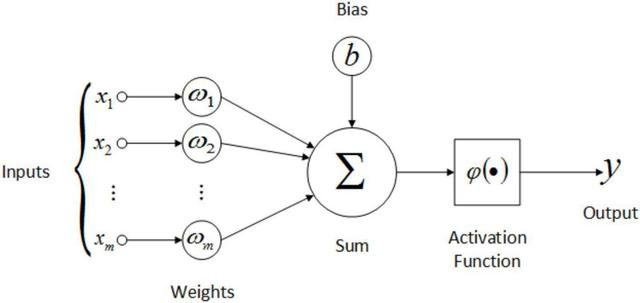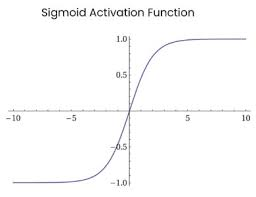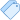July 11, 2020 Deepak Raj

### Introduction

The study of the human brain is thousands of years old. The first step toward artificial neural networks came in 1943 when Warren McCulloch, a neurophysiologist, and a young mathematician, Walter Pitts, wrote a paper on how neurons might work. They modelled a simple neural network with electrical circuits.

### Perceptrons

Perceptron is a binary linear classification algorithm. It's a supervised type of machine learning and the simplest form of neural network. It's a base for neural networks. So, if you want to know how neural network works, learn how perception works.

### Component of Perceptrons

These are the major components of perceptrons.

1. Input Value
2. Weight and Bias
3. Net Sum
4. Activation Function

Input Value:- Input Values are the feature of the Data set.

Weight and Bias:- Weights are the values that are computed over the time of training the model. Initial we start the value of weights with some initial value and these values get updated for each training basis of the error function.Bias is like the intercept added in a linear equation. It is an additional parameter in the Neural Network which is used to adjust the output along with the weighted sum of the inputs to the neuron.It helps in training the model faster and with better quality.

Net Sum:- The net sum is the sum of the multiplication of input and weight.

$$Net Sum = Σ w_i. x_i$$

Activation Function:- Activation function is used to make neural network non-linear. It's limit the output between 0 and 1.Any comments or if you have any question, write it in the comment.tags: Neural network, machine learning, deep learning